#Julia Set Images

Math Lair Home > Topics > Julia Set Images

The Julia set is a type of fractal. More information about this set is available on my Julia set page. Here is a graphical representation of the Julia set where the real value of c is -0.755 and the imaginary value of c ranges between 0 and 0.15i: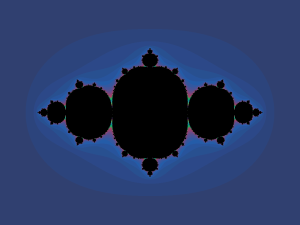c = -0.755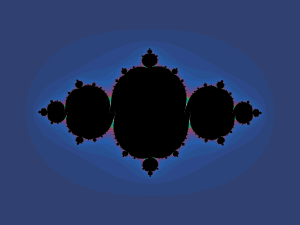c = -0.755 + 0.01i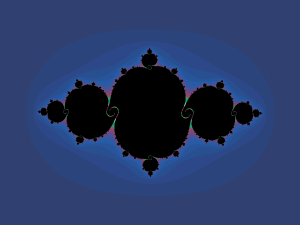c = -0.755 + 0.02ic = -0.755 + 0.03i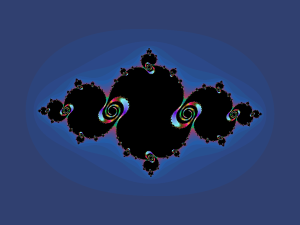c = -0.755 + 0.04ic = -0.755 + 0.05ic = -0.755 + 0.0525ic = -0.755 + 0.055ic = -0.755 + 0.575ic = -0.755 + 0.06ic = -0.755 + 0.07i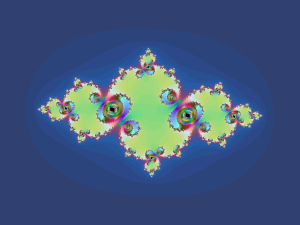c = -0.755 + 0.08ic = -0.755 + 0.1ic = -0.755 + 0.15i